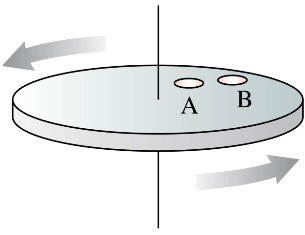# Problem: Two coins rotate on a turntable. The turntable is also speeding up. Coin B is twice as far from the axis as coin A.1. The angular velocity of coin B is ___ that of coin A.a. Four Times. b. twice c. same as d. half e. 1/42. The angular acceleration of coin B is ___ that of coin A. a. Four Times. b. twice c. same as d. half e. 1/4

###### FREE Expert Solution

In this problem, we looking into the effect of the radius on angular velocity and angular acceleration for the same rotational speed.

Angular velocity is the angle covered in one second:

$\overline{){\mathbf{\omega }}{\mathbf{=}}\frac{\mathbf{∆}\mathbf{\theta }}{\mathbf{∆}\mathbf{r}}}$

86% (209 ratings)###### Problem DetailsTwo coins rotate on a turntable. The turntable is also speeding up. Coin B is twice as far from the axis as coin A.

1. The angular velocity of coin B is ___ that of coin A.a. Four Times. b. twice c. same as d. half e. 1/4

2. The angular acceleration of coin B is ___ that of coin A. a. Four Times. b. twice c. same as d. half e. 1/4